# Area Moment of Inertia: Manual Calculation vs CAD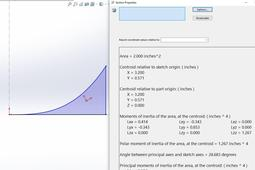Area Moment of Inertia: Manual Calculations Validated in CAD (SolidWorks)

1. ### Step 1: Area M of I by Integration

First, let's calculate the Area underneath the curve using Integration: A = ∫da; which for this area underneath the curve gives us 2.0 in^2.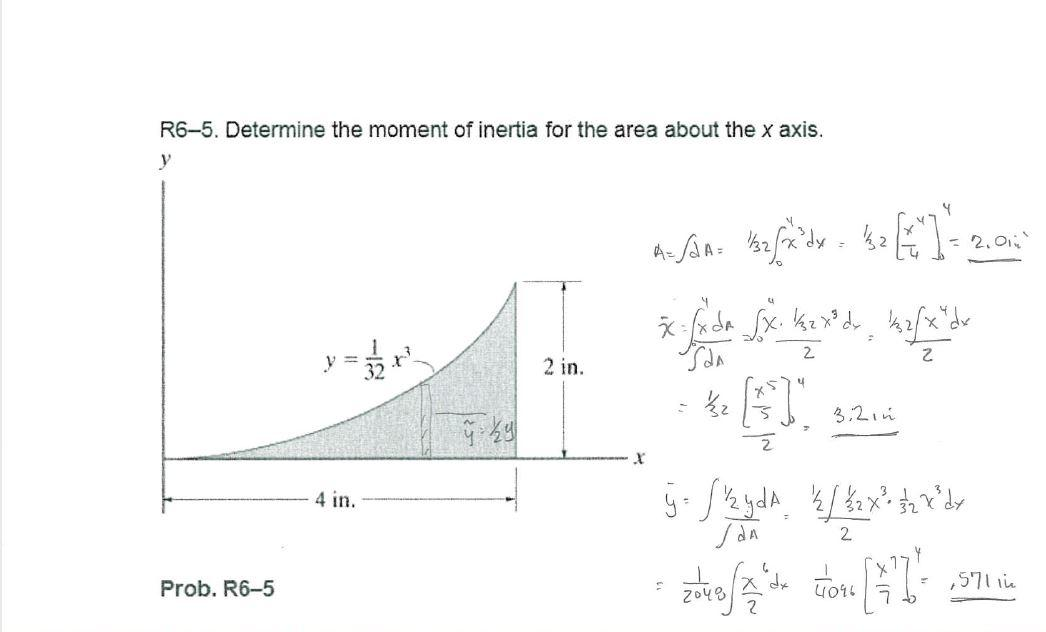Second, calculate the X-bar and y-bar or the centroid of the area.

x = ∫xda/∫da = 3.2 in.

y = ∫yda/∫da = .571 in.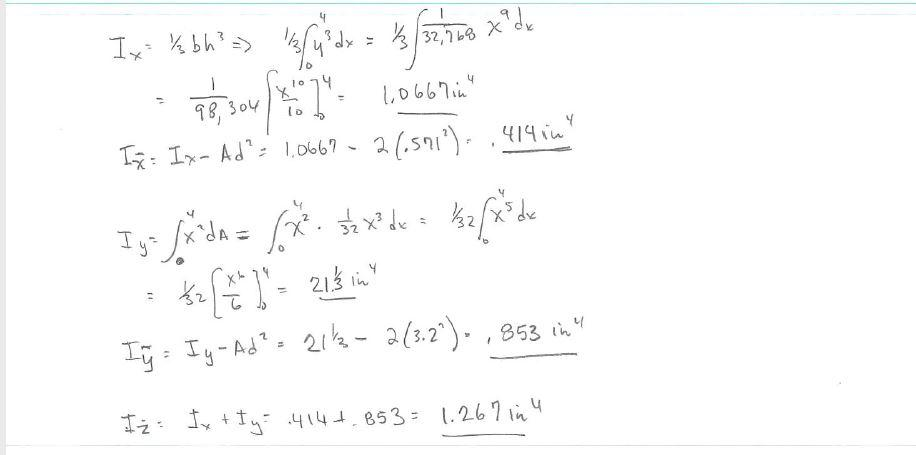2. ### Step 2: M of I about X-axis

We first calculate the Moment of Inertia about the X and Y axes with the equations shown using a vertical element. Then the Parallel Axis Theorem is used to move the Moment of Inertia to the Centrodial X-axis and Centrodial Y-axis.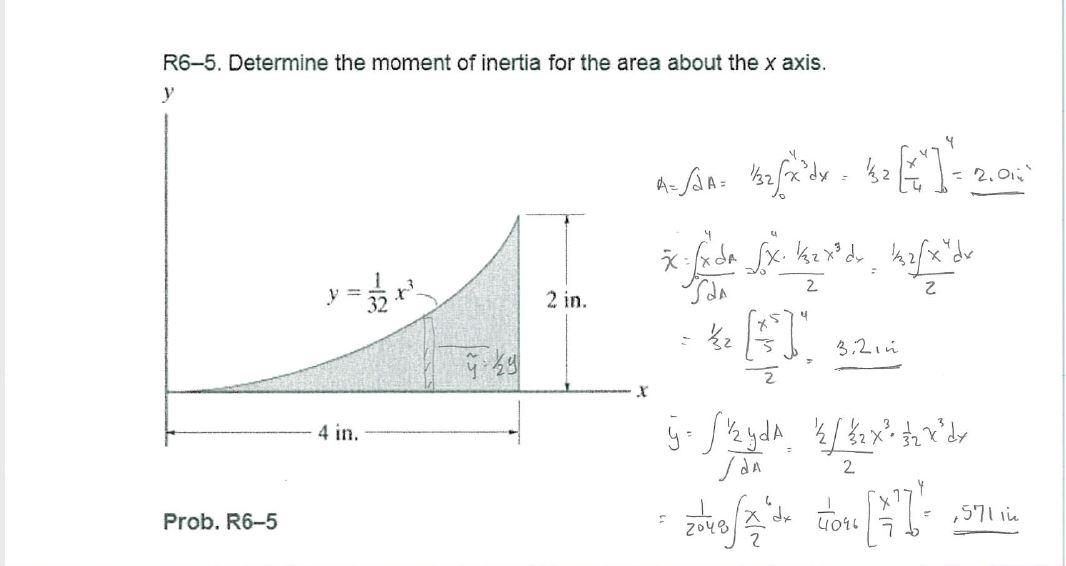Notice: that in the manual method for a function like this, for the Moment of Inertial, the value was first calculated with respect to the X-axis using Ixx = 1/3 bh^3. This is because the vertical element in Integration would have varying locations for the Centrodial x-axis but a constant x-axis. If a horizontal element was used, then we could use the standard Ixx= y^2da

3. ### Step 3: Sketch in function in CAD

In this case we are suing SolidWorks to create the curve.

a- Sketch on the X-Y Plane

b- Equation Driven Curve

c-For this function, notice the value of y = 1/32 x^3, so in SolidWorks we input these values as shown: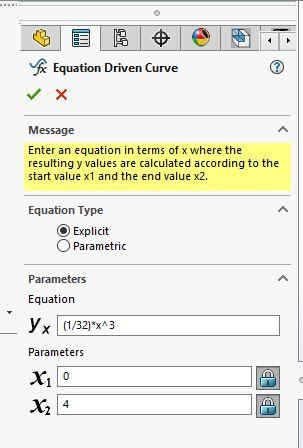Which gives us the function curve: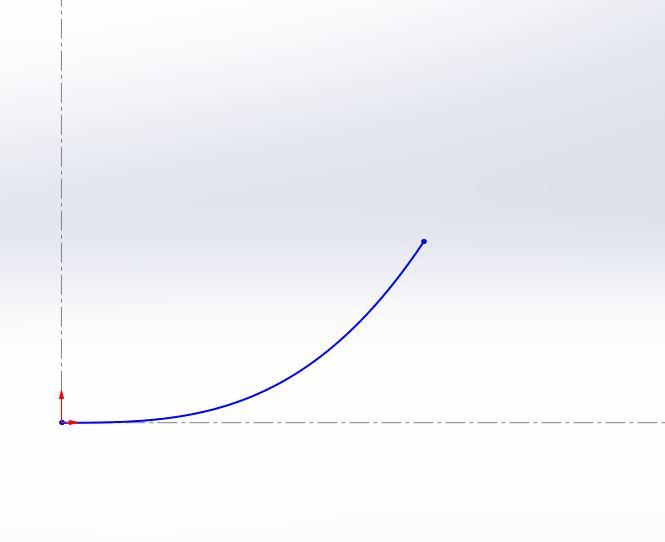The enclose the curve with vertical and horizontal lines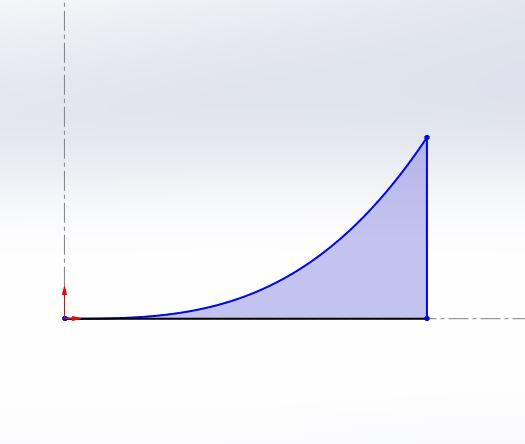4. ### Step 4:

Now, calculate the Section Properties of the Area;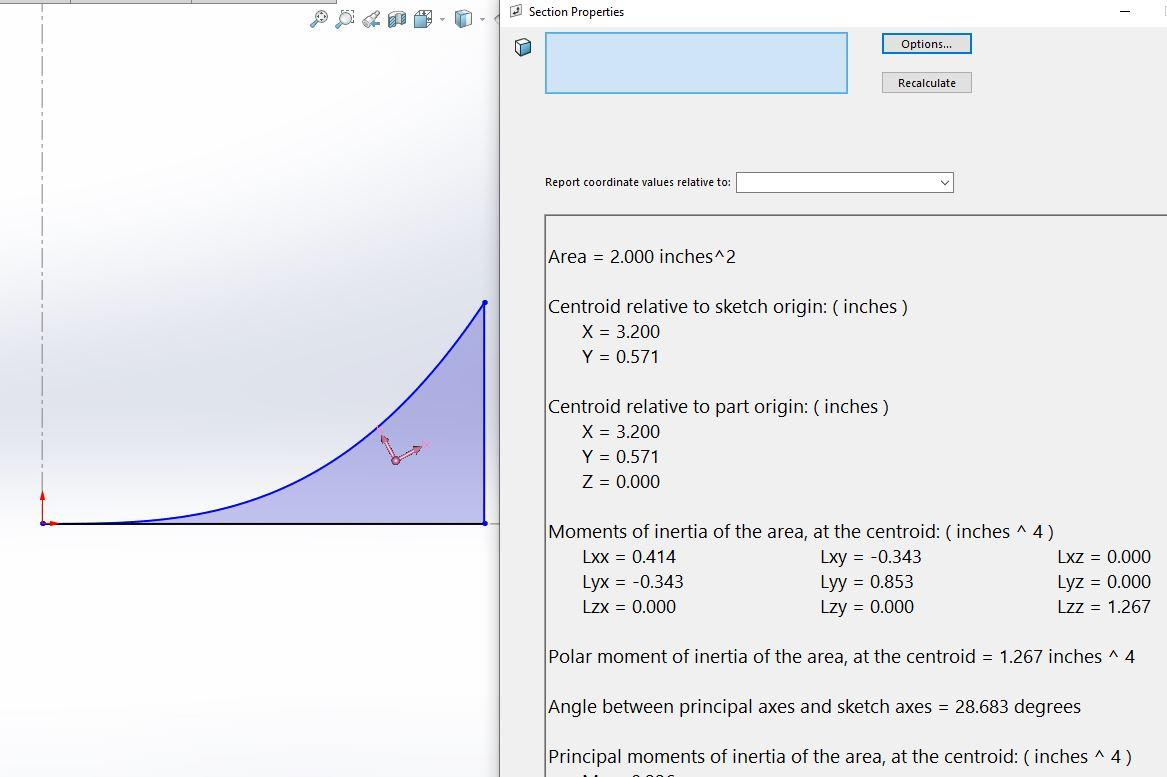The values match our manual calculations.

Area = 2.00 in^2

x-bar = 3.2 in

y-bar = .571 in

Ixx = .414 in^4

Iyy = .853 in^4

Izz = 1.267 in^4